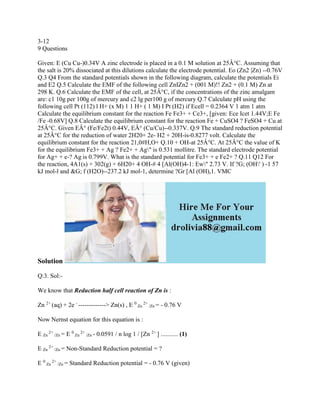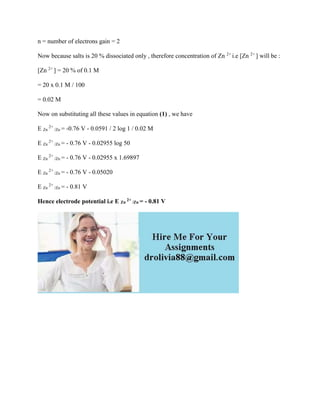Anúncio

# 3-12 9 Questions Given- E (Cu Cu-)0-34V A zinc electrode is placed in.docx

8 de Feb de 2023Próximos SlideShares1- Write out the half reactions- the net chemical reaction and the Eo.docx
Carregando em ... 3
1 de 2
Anúncio

### 3-12 9 Questions Given- E (Cu Cu-)0-34V A zinc electrode is placed in.docx

1. 3-12 9 Questions Given: E (Cu Cu-)0.34V A zinc electrode is placed in a 0.1 M solution at 25Â°C. Assuming that the salt is 20% dissociated at this dilutions calculate the electrode potential. Eo (Zn2 |Zn) --0.76V Q.3 Q4 From the standard potentials shown in the following diagram, calculate the potentials Ei and E2 Q.5 Calculate the EMF of the following cell ZnIZn2 + (001 M)!! Zn2 + (0.1 M) Zn at 298 K. Q.6 Calculate the EMF of the cell, at 25Â°C, if the concentrations of the zinc amalgam are: c1 10g per 100g of mercury and c2 lg per100 g of mercury Q.7 Calculate pH using the following cell Pt (112) l H+ (x M) 1 1 H+ ( 1 M) I Pt (H2) if Ecell = 0.2364 V 1 atm 1 atm Calculate the equilibrium constant for the reaction Fe Fe3+ + Ce3+, [given: Ece Icet 1.44V;E Fe /Fe -0.68V] Q.8 Calculate the equilibrium constant for the reaction Fe + CuSO4 ? FeSO4 + Cu at 25Â°C. Given EÂ° (Fe/Fe2t) 0.44V, EÂ° (Cu/Cu)--0.337V. Q.9 The standard reduction potential at 25Â°C for the reduction of water 2H20+ 2e- H2 + 20H-is-0.8277 volt. Calculate the equilibrium constant for the reaction 21,0#H,O+ Q.10 + OH-at 25Â°C. At 25Â°C the value of K for the equilibrium Fe3+ + Ag ? Fe2+ + Ag" is 0.531 mollitre. The standard electrode potential for Ag+ + e-? Ag is 0.799V. What is the standard potential for Fe3+ + e Fe2+ ? Q.11 Q12 For the reaction, 4A1(s) + 302(g) + 6H20+ 4 OH-# 4 [Al(OH)4-1: Ew" 2.73 V. If ?G; (OH' ) -1 57 kJ mol-l and &G; f (H2O)--237.2 kJ mol-1, determine ?Gr [Al (OH),1. VMC Solution Q:3. Sol:- We know that Reduction half cell reaction of Zn is : Zn 2+ (aq) + 2e - -------------> Zn(s) , E 0 Zn 2+ /Zn = - 0.76 V Now Nernst equation for this equation is : E Zn 2+ /Zn = E 0 Zn 2+ /Zn - 0.0591 / n log 1 / [Zn 2+ ] ........... (1) E Zn 2+ /Zn = Non-Standard Reduction potential = ? E 0 Zn 2+ /Zn = Standard Reduction potential = - 0.76 V (given)
2. n = number of electrons gain = 2 Now because salts is 20 % dissociated only , therefore concentration of Zn 2+ i.e [Zn 2+ ] will be : [Zn 2+ ] = 20 % of 0.1 M = 20 x 0.1 M / 100 = 0.02 M Now on substituting all these values in equation (1) , we have E Zn 2+ /Zn = -0.76 V - 0.0591 / 2 log 1 / 0.02 M E Zn 2+ /Zn = - 0.76 V - 0.02955 log 50 E Zn 2+ /Zn = - 0.76 V - 0.02955 x 1.69897 E Zn 2+ /Zn = - 0.76 V - 0.05020 E Zn 2+ /Zn = - 0.81 V Hence electrode potential i.e E Zn 2+ /Zn = - 0.81 V
Anúncio